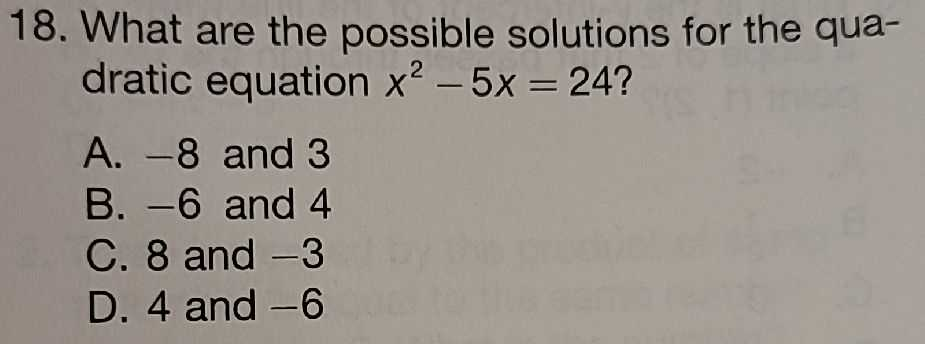### ¿Todavía tienes preguntas de matemáticas?

Pregunte a nuestros tutores expertos
Algebra
Pregunta18. What are the possible solutions for the qua- dratic equation $$x ^ { 2 } - 5 x = 24 ?$$

A. $$- 8$$ and $$3$$ B. $$- 6$$ and $$4$$ C. $$8$$ and $$- 3$$ D. $$4$$ and $$- 6$$